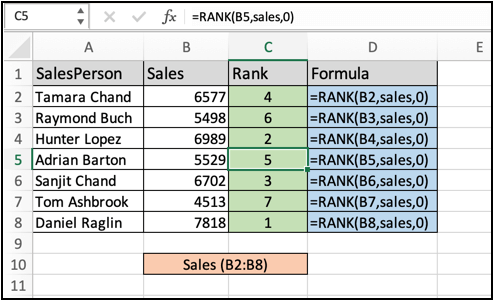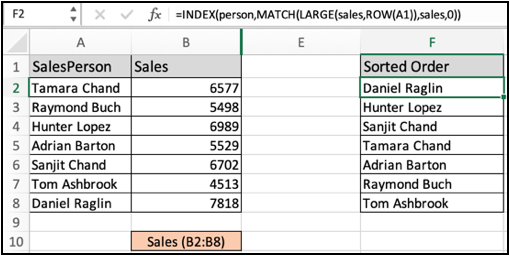# How to Rank Salespeople According to Sales Figure in ExcelIn this article, we will learn How to Rank Salespeople According to Sales Figure in Excel.

Scenario :

In excel, ranking is used as statistics to show a relation. Ranking numbers is a very common and useful appendage when worming sales data. For example finding the top salesperson and lowest salesperson in the department. For problems like these we use the RANK formula in excel explained below with an example.

Rank formula in Excel

Rank function in excel takes two arguments first is the value itself and second is the array within ranking required. Learn how to use the RANK function in excel below.

Excel Rank formula

 =RANK(value, array, 0)

value : value to rank

array : array in which to rank

Example :

All of these might be confusing to understand. Let's understand how to use the function using an example. Here we have some sales data to handle. First we rank all the values using the rank formula in excel.

Use the formula:

 =RANK(B2,sales,0)

Here sales is fixed array B2:B6Copy and paste the formula to other cells using Ctrl + D. As you can see in the above image all the ranks with respective formulas. Now we can also sort the salesperson based on their respective rank or sales figure in excel.

Sort sales person using the sales figure

Sorting in excel can be done by Sort and filter option and can also be done using excel functions. Use the below formula to understand the new sorted sales person column.

Use the formula:

 =INDEX(person,MATCH(LARGE(sales,ROW(A1)),sales,0))

Here sales is fixed array B2:B6 and person is fixed array A2:A6As you can see now you have another sorted column using excel functions. Now You have learnt how to sort columns based on the other columns.

Here are all the observational notes using the formula in Excel
Notes :

1. Using the Sort & Filter option is the most common and easy practice in excel.
2. You can sort numbers ascending or descending. Or sort text values a-z (alphabetically) or z-a (reverse alphabetically).

Hope this article about How to Rank Salespeople According to Sales Figure in Excel is explanatory. Find more articles on calculating values and related Excel formulas here. If you liked our blogs, share it with your friends on Facebook. And also you can follow us on Twitter and Facebook. We would love to hear from you, do let us know how we can improve, complement or innovate our work and make it better for you. Write to us at info@exceltip.com.

Related Articles :

How to use Shortcut Keys for Sort & filter in Excel : Use Ctrl + Shift + L from keyboard to apply sort and filter on table in Excel.

The SORT Function in Excel 365 (new version) : The SORT function  returns the sorted array by the given column number in the array. It also works on horizontal data.

Sort numbers using Excel SMALL Function : To sort numbers using formula in Excel 2016 and older, you can use the SMALL function with other helping functions.

Sort Numeric Values with Excel RANK Function : To sort the numeric values we can use the Rank function. The formula is

Excel Formula to Sort Text : To sort text values using formula in excel we simply use the COUNTIF function. Here is the formula

Excel Formula to Extract Unique Values From a List : To find all unique values from a list in Excel 2016 and older versions, we use this simple formula.

UNIQUE Function for Excel 2016 : The Excel 2016 does not provide the UNIQUE function but we can create one. This UDF UNIQUE function returns all unique values from the list.

How To Count Unique Values in Excel With Criteria : To count unique values in excel with criteria we use a combination of functions. The FREQUENCY function is core of this formula

Popular Articles :